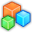# Microsoft Small BasicProgram Listing: GSX344
' This is a program to reproduce following known issue of Small Basic v1.0.
' A shape moving over a stationary mouse causes MouseMove event.
CRLF = Text.GetCharacter(13) + Text.GetCharacter(10)
txt = "This program changes background color "
txt = txt + "when mouse move event occurs." + CRLF
txt = txt + "Place mouse pointer on one of circles. "
txt = txt + "These circles are only one ellipce created "
txt = txt + "by Shapes object. This causes mouse move event."
GraphicsWindow.DrawBoundText(10, 10, 600, txt)
x = "1=100;2=500;3=500;4=100;"
y = "1=100;2=100;3=300;4=300;"
max = Array.GetItemCount(x)